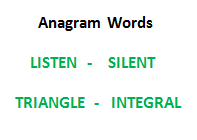Open in App
Not now

# Javascript Program To Check Whether Two Strings Are Anagram Of Each Other

• Last Updated : 25 Aug, 2022

Write a function to check whether two given strings are anagram of each other or not. An anagram of a string is another string that contains the same characters, only the order of characters can be different. For example, “abcd” and “dabc” are an anagram of each other.## We strongly recommend that you click here and practice it, before moving on to the solution.

Method 1 (Use Sorting):

1. Sort both strings
2. Compare the sorted strings

Below is the implementation of the above idea:

## Javascript

 ``

Output:

`The two strings are not anagram of each other`

Time Complexity: O(nLogn)

Auxiliary space: O(1).

Method 2 (Count characters):
This method assumes that the set of possible characters in both strings is small. In the following implementation, it is assumed that the characters are stored using 8 bit and there can be 256 possible characters.

1. Create count arrays of size 256 for both strings. Initialize all values in count arrays as 0.
2. Iterate through every character of both strings and increment the count of character in the corresponding count arrays.
3. Compare count arrays. If both count arrays are same, then return true.

Below is the implementation of the above idea:

## Javascript

 ``

Output:

`The two strings are anagram of each other`

Time Complexity: O(n)

Auxiliary space: O(n).

Method 3 (count characters using one array):
The above implementation can be further to use only one count array instead of two. We can increment the value in count array for characters in str1 and decrement for characters in str2. Finally, if all count values are 0, then the two strings are anagram of each other. Thanks to Ace for suggesting this optimization.

## Javascript

 ``

Output:

`The two strings are anagram of each other`

Time Complexity: O(n)

Auxiliary space: O(n).

Method 4 (Using Map()):

We can optimize the space complexity of the above method by using HashMap instead of initializing 256 characters array. So in this approach, we will first count the occurrence of each unique character with the help of HashMap for the first string. Then we will reduce the count of each character while we encounter them in the second string. Finally, if the count of each character in the hash map is 0 then it means both strings are anagrams else not.

Below is the code for the above approach.

## Javascript

 ``

Time Complexity: O(n)

Auxiliary space: O(n) because it is using HashMap

Please suggest if someone has a better solution which is more efficient in terms of space and time.